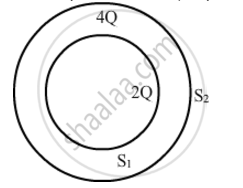# Consider Two Hollow Concentric Spheres, S1 and S2, Enclosing Charges 2q and 4q Respectively as Shown in the Figure. (I) Find Out the Ratio of the Electric Flux Through Them - Physics

Consider two hollow concentric spheres, S1 and S2, enclosing charges 2Q and 4Q respectively as shown in the figure. (i) Find out the ratio of the electric flux through them. (ii) How will the electric flux through the sphere S1 change if a medium of dielectric constant 'εr' is introduced in the space inside S1 in place of air ? Deduce the necessary expression#### Solution

(i) Charge enclosed by sphere S1 = 2Q

By Gauss law, electric flux through sphere S1 is

phi_1=(2Q)/in_0

Charge enclosed by sphere S2 = 2Q+4Q=6Q

:.phi_2=(6Q)/in_0

The ratio of the electric flux is

phi_1/phi_2=((2Q)/in_0)/((6Q)/in_0)=2/6=1/3

(ii) For sphere S1, the electric flux is

phi'=(2q)/in_r

:.(phi')/phi_1=in_0/in_r

=>phi'=phi_1.in_0/in_r

∵ εr ε0

`:.phi'

Therefore, the electric flux through the sphere S1 decreases with the introduction of the dielectric inside it.

Concept: Electric Flux
Is there an error in this question or solution?